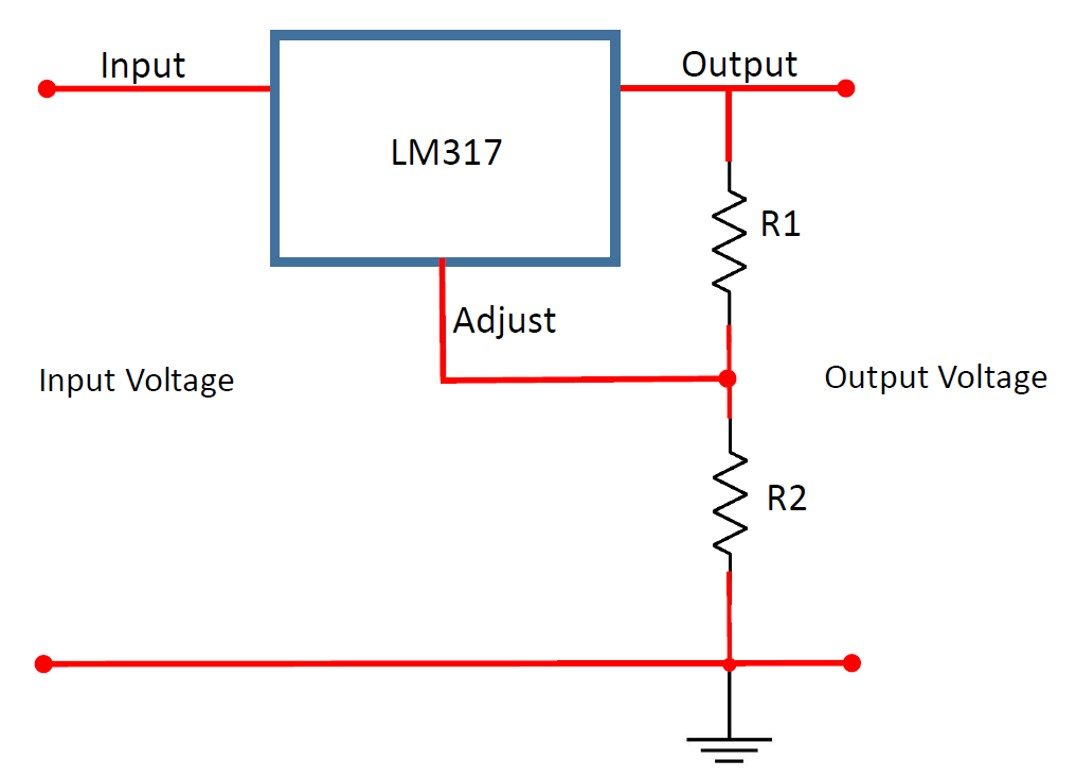# LM317 Calculator

Created by Purnima Singh, PhD
Reviewed by Steven Wooding
Last updated: Apr 06, 2022

If you want to know which external resistors to choose to get your desired output voltage from the LM317 voltage regulator, the LM317 calculator is the only tool you need.

Whether you are planning to repair one of your electronic devices or just want to learn the basics of electronics, you have stumbled into the right place. Read on to know what the LM317 voltage regulator is and how to calculate the required resistances to get your target output voltage. You will also find an LM317 circuit diagram and some common applications of the LM317 regulator.

## What is a LM317?

A LM317 is an adjustable DC voltage regulator. It can provide a steady output voltage even when there are fluctuations in the input power supply.
The regulator can supply an output current in the range of 0.01-1.5 A and a positive output voltage between 1.25 V and 37 V.

As you can see from the pinout of LM317 in figure 1, it is a three-terminal device. The three pins are:

• Input terminal;
• Output terminal; andFig. 1: The pinout of LM317 in a TO-220 package with the 1.25 voltage reference marked. (Source: wikimedia.org)

The input pin accepts the supply voltage and the output pin gives the regulated output voltage signal. The device can operate optimally as long as the difference between the input and output voltages lies in the range 3-40 V.
The adjust pin is connected to a resistor divider arrangement that uses two external resistors, R1 and R2 (see figure 2), to set the desired output voltage.

## How to adjust LM317 voltage regulator output voltage?

A LM317 is very easy to use, and we can vary the output voltage using a simple voltage divider arrangement consisting of two resistors, R1 and R2, as shown in the LM317 circuit diagram of figure 2.

The formula for calculating output voltage of LM317 is:

VOut = Vref * (1 + R2 / R1) + (IADJ * R2)

where:

• Vref — Voltage difference between the output and adjustment terminal of LM317. It has a constant value of 1.25 V; and
• IADJ — Current that flows through the adjust pin. The typical value of IADJ is 50 µA and can be neglected in calculations.

Hence, we can write a simplified form for the above equation as:

VOut = 1.25 * (1 + R2 / R1)

You can set the regulator to give a constant output voltage from 1.25 V to 37 V by choosing the appropriate resistors.

## How to use the LM317 calculator?

Let us see how to use the LM317 calculator to calculate the output voltage. Let R1 = 240 Ω and R2 = 1200 Ω.

1. Enter the values of R1 = 240 Ω and R2 = 1200 Ω.
2. The LM317 output voltage calculator will display the output voltage V = 7.5 V.
3. You can also use this LM317 calculator to calculate the value of R1 (or R2) by supplying the values of V and R2 (or R1).

## Applications of LM317

LM317 voltage regulators are widely used in electronic devices that use a regulated DC power supply. Some common devices that use LM317 as a variable voltage regulator are:

• Desktop PCs;
• Ethernet switches;
• Power banks;
• Hydraulic valves; and
• Refrigerators.

## FAQ

### How does LM317 work?

A LM317 is a voltage regulator that can supply variable output voltage. The working principle of the LM317 is very simple. It uses a voltage divider, formed by using two external resistors connected in series. The output voltage is adjusted by varying the resistor values.

### What is a LM317 used for?

The LM317 is commonly used as:

• A voltage regulator in electronic devices to provide a stable output voltage.
• A precision current regulator to provide constant current.
• In designing battery charger circuits and variable power supplies.

### What is the maximum input voltage of a LM317?

The maximum input voltage of LM317 is 40 V. The LM317 can operate as long as the difference between its input voltage and the desired output voltage is in the range 3-40 V.

### What is the output voltage of a LM317?

The LM317 can provide an adjustable output voltage between 1.25 V and 37 V. It can supply current up to 1.5 A.

Purnima Singh, PhD
Resistor (R1)
Ω
Resistor (R2)
Ω
Output voltage
VPeople also viewed…

### Black Hole Collision Calculator

The Black Hole Collision Calculator lets you see the effects of a black hole collision, as well as revealing some of the mysteries of black holes, come on in and enjoy!

### Eggs

Quantum physicist's take on boiling a perfect egg.

### Fret